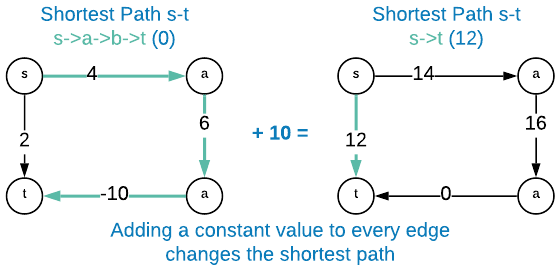# Dijkstra's Shortest Path (Naive)

Dijkstra’s shortest path algorithm calculates the shortest distance between vertices in a weighted graph with non-negative edge weights. This is without a doubt one of the greatest hits of algorithms with countless applications. Those wishing to excel in computer science should take extra car to ensure they fully understand this.

A common error is to assume that the non-negative edge weight constraint is circumventable by increasing the value of each edge weight by the absolute value of the smallest edge weight ($\mid\min{e_w\in E}\mid$). The flaw in this assumption is best demonstrated with an example.As the graphic above illustrates, adding a constant value to each edge weight results in altering the shortest path.

The algorithm as shown in the this section is a naive implementation. Each iteration of the main while loop requires a scan over all edges to find the one with the minimum distance. While this runtime isn’t horrible, it’s possible to do much better using the implementation in the next section.

## Asymptotic Complexity

$O(mn)$

## Pseudo Code

dijkstra:
G = input graph with distance of each vertex set to infinity
v = starting vertex
side effects: marks all vertices with the shortest distance from the starting
vertex

S = empty array

v.distance = 0

while true:
u = find_min(G, S)
if u is NULL or u.distance == infinity:
break

find_min:
G = input graph
S = array containing all conquered vertices

v = NULL

for each vertex in S:
for each edge in vertex:
if edge.head is contained in S:
continue

distance = edge.tail.distance + edge.weight